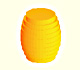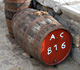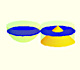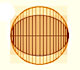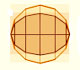Kepler wrote a book in 1615 about areas and volumes of surfaces and solids: Nova stereometria doliorum vinariorum (New solid geometry of wine barrels). In this book Kepler thought of the sphere as being composed of an infinite number of infinitesimal pyramids or cones each having its vertex at the center of the sphere and whose bases made up the surface of the sphere.

The volume of a pyramid or a cone is one-third the product of the area of the base and the altitude (in this case the altitude is the radius of the sphere). Then Kepler was able to deduce the relation between the volume of the sphere and its surface:

Then, if we know the volume of the sphere (as Archimedes knew more than 2200 years before us):

we can deduce the area of the surface of the sphere:

Or viceversa, if we know the surface we can deduce the volume of the sphere.

This is one example of a theorem about the sphere in one original Kepler's Nova stereometria doliorum vinariorum. We can read this page, and the full book, in The Posner Memorial Collection,Carnegie Mellon University Libraries.

A translation could be: The surface area of a sphere is four times the area of a great circle of the sphere.

REFERENCES

Felix Klein - Elementary Mathematics from an Advanced Standpoint. Arithmetic, Algebra, Analysis (p. 209) - Dover Publications
Carl B. Boyer - The History of the Calculus and its Conceptual Development (p. 108)-- Dover Publications
C.H. Edwards - The Historical Development of the Calculus (p. 102)- Springer-Verlag